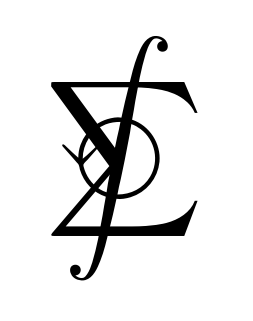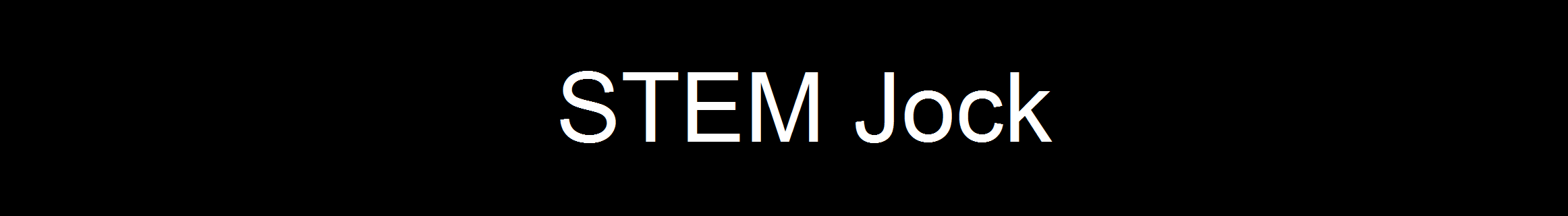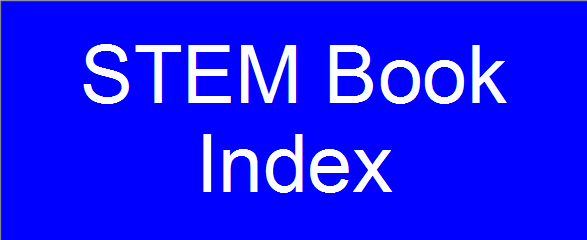On this webpage you will find my solutions to Chapters 1-5 of the eighth edition of "Calculus: Early Transcendentals" by James Stewart. Solutions to Chapters 6-11 are here, and solutions to Chapters 12-17 are here. Here is a link to the book's page on amazon.com. If you find my work useful, please consider making a donation. Thank you.

• Chapter 1: Functions and Models
• Section 1.1: Four Ways to Represent a Function
• Section 1.2: Mathematical Models: A Catalog of Essential Functions
• Section 1.3: New Functions from Old Functions
• Section 1.4: Exponential Functions
• Section 1.5: Inverse Functions and Logarithms

• Chapter 2: Limits and Differentiation
• Section 2.1: The Tangent and Velocity Problems
• Section 2.2: The Limit of a Function
• Section 2.3: Calculating Limits Using the Limit Laws
• Section 2.4: The Precise Definition of a Limit
• Section 2.5: Continuity
• Section 2.6: Limits at Infinity; Horizontal Asymptotes
• Section 2.7: Derivatives and Rates of Change
• Section 2.8: The Derivative as a Function

• Chapter 3: Differentiation Rules
• Section 3.1: Derivatives of Polynomials and Exponential Functions
• Section 3.2: The Product and Quotient Rules
• Section 3.3: Derivatives of Trigonometric Functions
• Section 3.4: The Chain Rule
• Section 3.5: Implicit Differentiation
• Section 3.6: Derivatives of Logarithmic Functions
• Section 3.7: Rates of Change in the Natural and Social Sciences
• Section 3.8: Exponential Growth and Decay
• Section 3.9: Related Rates
• Section 3.10: Linear Approximations and Differentials
• Section 3.11: Hyperbolic Functions

• Chapter 4: Applications of Differentiation
• Section 4.1: Maximum and Minimum Values
• Section 4.2: The Mean Value Theorem
• Section 4.3: How Derivatives Affect the Shape of a Graph
• Section 4.4: Indeterminate Forms and l'Hospital's Rule
• Section 4.5: Summary of Curve Sketching
• Section 4.6: Graphing with Calculus and Calculators
• Section 4.7: Optimization Problems
• Section 4.8: Newton's Method
• Section 4.9: Antiderivatives

• Chapter 5: Integrals
• Section 5.1: Areas and Distances
• Section 5.2: The Definite Integral
• Section 5.3: The Fundamental Theorem of Calculus
• Section 5.4: Indefinite Integrals and the Net Change Theorem
• Section 5.5: The Substitution Rule
• Chapter 6: Applications of Integration
• Section 6.1: Areas Between Curves
• Section 6.2: Volumes
• Section 6.3: Volumes by Cylindrical Shells
• Section 6.4: Work
• Section 6.5: Average Value of a Function

• Chapter 7: Techniques of Integration
• Section 7.1: Integration by Parts
• Section 7.2: Trigonometric Integrals
• Section 7.3: Trigonometric Substitution
• Section 7.4: Integration of Rational Functions by Partial Fractions
• Section 7.5: Strategy for Integration
• Section 7.6: Integration Using Tables and Computer Algebra Systems
• Section 7.7: Approximate Integration
• Section 7.8: Improper Integrals

• Chapter 8: Further Applications of Integration
• Section 8.1: Arc Length
• Section 8.2: Area of a Surface of Revolution
• Section 8.3: Applications to Physics and Engineering
• Section 8.4: Applications to Economics and Biology
• Section 8.5: Probability

• Chapter 9: Differential Equations
• Section 9.1: Modeling with Differential Equations
• Section 9.2: Direction Fields and Euler's Method
• Section 9.3: Separable Equations
• Section 9.4: Models for Population Growth
• Section 9.5: Linear Equations
• Section 9.6: Predator-Prey Systems

• Chapter 10: Parametric Equations and Polar Coordinates
• Section 10.1: Curves Defined by Parametric Equations
• Section 10.2: Calculus with Parametric Curves
• Section 10.3: Polar Coordinates
• Section 10.4: Areas and Lengths in Polar Coordinates
• Section 10.5: Conic Sections
• Section 10.6: Conic Sections in Polar Coordinates

• Chapter 11: Infinite Series
• Section 11.1: Sequences
• Section 11.2: Series
• Section 11.3: The Integral Test and Estimates of Sums
• Section 11.4: The Comparison Tests
• Section 11.5: Alternating Series
• Section 11.6: Absolute Convergence and the Ratio and Root Tests
• Section 11.7: Strategy for Testing Series
• Section 11.8: Power Series
• Section 11.9: Representations of Functions as Power Series
• Section 11.10: Taylor and Maclaurin Series
• Section 11.11: Applications of Taylor Polynomials
• Chapter 12: Vectors and the Geometry of Space
• Section 12.1: Three-Dimensional Coordinate Systems
• Section 12.2: Vectors
• Section 12.3: The Dot Product
• Section 12.4: The Cross Product
• Section 12.5: Equations of Lines and Planes
• Section 12.6: Cylinders and Quadric Surfaces

• Chapter 13: Vector Functions
• Section 13.1: Vector Functions and Space Curves
• Section 13.2: Derivatives and Integrals of Vector Functions
• Section 13.3: Arc Length and Curvature
• Section 13.4: Motion in Space: Velocity and Acceleration

• Chapter 14: Partial Derivatives
• Section 14.1: Functions of Several Variables
• Section 14.2: Limits and Continuity
• Section 14.3: Partial Derivatives
• Section 14.4: Tangent Planes and Linear Approximations
• Section 14.5: The Chain Rule
• Section 14.6: Directional Derivatives and the Gradient Vector
• Section 14.7: Maximum and Minimum Values
• Section 14.8: Lagrange Multipliers

• Chapter 15: Multiple Integrals
• Section 15.1: Double Integrals over Rectangles
• Section 15.2: Double Integrals over General Regions
• Section 15.3: Double Integrals in Polar Coordinates
• Section 15.4: Applications of Double Integrals
• Section 15.5: Surface Area
• Section 15.6: Triple Integrals
• Section 15.7: Triple Integrals in Cylindrical Coordinates
• Section 15.8: Triple Integrals in Spherical Coordinates
• Section 15.9: Change of Variables in Multiple Integrals

• Chapter 16: Vector Calculus
• Section 16.1: Vector Fields
• Section 16.2: Line Integrals
• Section 16.3: The Fundamental Theorem for Line Integrals
• Section 16.4: Green's Theorem
• Section 16.5: Curl and Divergence
• Section 16.6: Parametric Surfaces and Their Areas
• Section 16.7: Surface Integrals
• Section 16.8: Stokes' Theorem
• Section 16.9: The Divergence Theorem

• Chapter 17: Second-Order Differential Equations
• Section 17.1: Second-Order Linear Equations
• Section 17.2: Nonhomogeneous Linear Equations
• Section 17.3: Applications of Second-Order Differential Equations
• Section 17.4: Series Solutions
Section 2.1 Section 2.2 Section 2.3
Exercise 1 Exercise 1 Exercise 23 Exercise 45 Exercise 1 Exercise 23 Exercise 45
Exercise 2 Exercise 2 Exercise 24 Exercise 46 Exercise 2 Exercise 24 Exercise 46
Exercise 3 Exercise 3 Exercise 25 Exercise 47 Exercise 3 Exercise 25 Exercise 47
Exercise 4 Exercise 4 Exercise 26 Exercise 48 Exercise 4 Exercise 26 Exercise 48
Exercise 5 Exercise 5 Exercise 27 Exercise 49 Exercise 5 Exercise 27 Exercise 49
Exercise 6 Exercise 6 Exercise 28 Exercise 50 Exercise 6 Exercise 28 Exercise 50
Exercise 7 Exercise 7 Exercise 29 Exercise 51 Exercise 7 Exercise 29 Exercise 51
Exercise 8 Exercise 8 Exercise 30 Exercise 52 Exercise 8 Exercise 30 Exercise 52
Exercise 9 Exercise 9 Exercise 31 Exercise 53 Exercise 9 Exercise 31 Exercise 53
Exercise 10 Exercise 32 Exercise 54 Exercise 10 Exercise 32 Exercise 54
Exercise 11 Exercise 33 Exercise 55 Exercise 11 Exercise 33 Exercise 55
Exercise 12 Exercise 34 Exercise 12 Exercise 34 Exercise 56
Exercise 13 Exercise 35 Exercise 13 Exercise 35 Exercise 57
Exercise 14 Exercise 36 Exercise 14 Exercise 36 Exercise 58
Exercise 15 Exercise 37 Exercise 15 Exercise 37 Exercise 59
Exercise 16 Exercise 38 Exercise 16 Exercise 38 Exercise 60
Exercise 17 Exercise 39 Exercise 17 Exercise 39 Exercise 61
Exercise 18 Exercise 40 Exercise 18 Exercise 40 Exercise 62
Exercise 19 Exercise 41 Exercise 19 Exercise 41 Exercise 63
Exercise 20 Exercise 42 Exercise 20 Exercise 42 Exercise 64
Exercise 21 Exercise 43 Exercise 21 Exercise 43 Exercise 65
Exercise 22 Exercise 44 Exercise 22 Exercise 44 Exercise 66